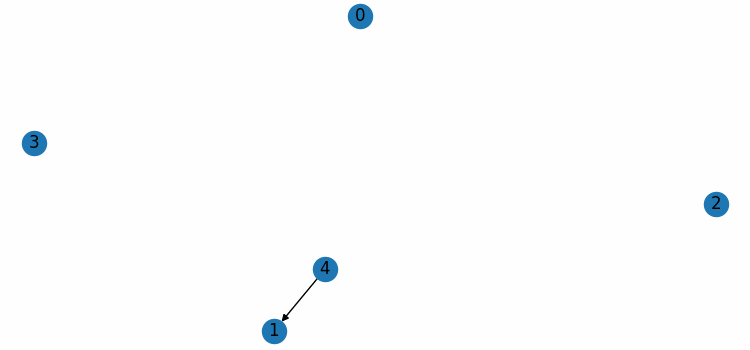# How to use an update function to animate a NetworkX graph in Matplotlib?

To use an update function to animate a NetworkX graph in Matplotlib, we can take the following steps −

• Set the figure size and adjust the padding between and around the subplots.
• Create a new figure or activate an existing figure using figure() method.
• Initialize a graph with edges, name, and graph attributes.
• Draw the graph G with Matplotlib.
• Use FuncAnimation() class to make an animation by repeatedly calling a function, animate.
• Function animate clears the current figure, generate two random numbers, and draws the edges between them.
• To display the figure, use show() method.

## Example

from matplotlib import pyplot as plt, animation
import networkx as nx
import random

plt.rcParams["figure.figsize"] = [7.50, 3.50]
plt.rcParams["figure.autolayout"] = True

fig = plt.figure()

G = nx.DiGraph()

nx.draw(G, with_labels=True)

def animate(frame):
fig.clear()
num1 = random.randint(0, 4)
num2 = random.randint(0, 4)
plt.show()